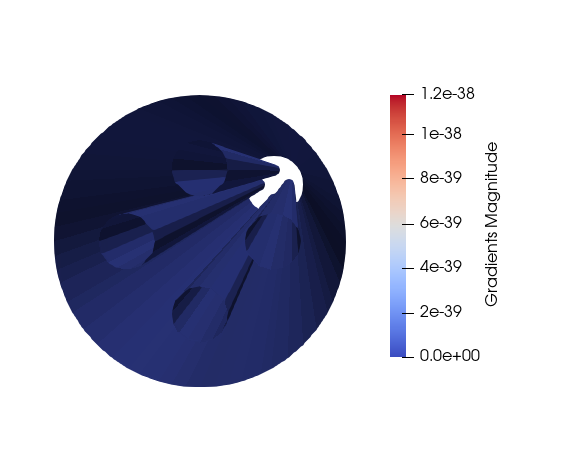# Gradient of unstructured datasets in paraview giving zero values at the walls.

Hi everyone,

Actually I am doing LES simulation in openfoam and I want to know the shear stress at the walls of my geometry. The geometry is quite a simple one with a cylinder and some internal pipes as shown in the attachment. Bounday condition at cylinder wall and internal pipe walls is zero velocity.

I have been researching a lot to figure out how to calculate shear stress on the walls and finally came to know that I should first find the gradient on the surface and then create surface normals and do the dot product but it seems out that gradient on my wall is always compute"d zero so the computations give me zero value. Can someone hemp me with this, please? I Have been stuck on this for quite a long time now.

Looking forward for some response;I don’t think anyone can answer your question without taking a look at your mesh (not just a screen shot). I can say that the `Gradient` filter can generally give non-zero values at the boundary of a mesh.

Just to verify, you are computing the gradient before extracting the surface, correct? If you attempt to run the gradient on the surface mesh, you will get the wrong answer.

This is somewhat of a guess but is OpenFOAM computing cell-centered values for the velocity? When this is done ParaView doesn’t have any idea about no-slip boundary conditions and the velocity doesn’t get properly projected onto the boundary for gradient calculations. I would suggest trying to get OpenFOAM to perform this type of calculation and save those results since it knows much more about the numerical schemes used in the calculation.

Actually, I have recently started playing around with Paraview so I am not sure if extracting the surface is important. From my understanding, I have patches defined from my simulation in openfoam, which are the cylinder wall and the walls of the internal pipes. I am actually selecting only these surfaces and not the internal mesh when doing the post-processing, since I am only interested in these surfaces. Following this, I am using a gradient filter on my velocity and then the surface normals.

From my knowledge, OpenFoam uses a finite volume method so it is calculating velocity at the cell centroid. I am not sure if one can change the way it does because I think it is how finite volume works, it calculates and stores data at the cell centroids. And the problem is that I am not sure how to perform shear stress calculations in openfoam. I have looked at various places but they are mostly for RANS simulations and for LES, the solver does not have this utility of calculating the shear stress calculations. I might be wrong but I am not able to find any kind of help for a few daysOK. It sounds like you are first extracting the external mesh (either in OpenFOAM or ParaView) and then performing the gradient. That’s just not going to do what you want. You started by saying that the velocity is zero on these boundaries. So, you have a surface with zero velocity everywhere and take the gradient. The gradient is also obviously 0. Even if it was not, when you take the gradient of a surface, the gradient is going to be tangent to the surface, which is useless if you want to take the dot product with the normal.

You are going to have to take the gradient of the whole mesh (or at least all cells incident on the boundary) and then extract the surface, find the normals, and do the dot product.

As @Andy_Bauer said, if you can finesse OpenFOAM to compute the gradient for you, that would probably be most accurate. But if you are OK with an approximation, loading the whole mesh into ParaView should work.

1 Like

Thanks a lot, guys. I understand,d the problem now. I will try to follow the same but extremely happy to receive the help. Really appreciate it.